• dwa算法
千次阅读
2022-04-04 16:17:59人工智能 matlab
更多相关内容
• Astar算法和DWA算法的结合实现 main.py：文件可以通过Astar算法实现两点间的路径规划 dwa.py：文件在main.py文件的基础上增加了dwa窗口动态算法，可以实现小车在运行过程中的避障功能 Vplanner.py：dwa算法实现 ...
• 路径规划DWA算法精简版，python matplotlib库绘制实时运动信息，注释齐全，简单易懂
• 【路径规划】基于改进动态窗口DWA算法机器人静态避障matlab源码
• DWA算法验证.rar,DWA算法验证,DWA算法验证截图.png,DWA算法matlab代码.txt,dwa_planner_ros1.cpp.pdf,DWA算法matlab代码.pdf
• 【路径规划】基于matlab DWA算法机器人局部避障路径规划【含Matlab源码 890期】.zip
• DWA算法（dynamicwindowapproach）是移动机器人在运动模型下推算（v,w）对应的轨迹，确定速度采样空间或者说是动态窗口（三种限制）；在速度空间（v,w）中采样多组速度，并模拟这些速度在一定时间内的运动轨迹，通过...

## 一、算法概述

DWA算法（dynamic window approach）是移动机器人在运动模型下推算（v,w）对应的轨迹，确定速度采样空间或者说是动态窗口（三种限制）；在速度空间（v,w）中采样多组速度，并模拟这些速度在一定时间内的运动轨迹，通过一个评价函数对这些轨迹打分，选取最优的轨迹来驱动机器人运动。

## 二、算法原理

### 1、运动学模型

**（1）非全向运动：**只能前进和旋转（相邻时刻码盘采样，近似直线v*detaT）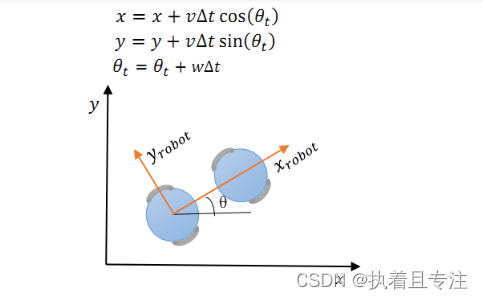**(2) 全向运动：**考虑x方向运动、y方向运动和旋转。将y轴移动距离投影世界坐标系上。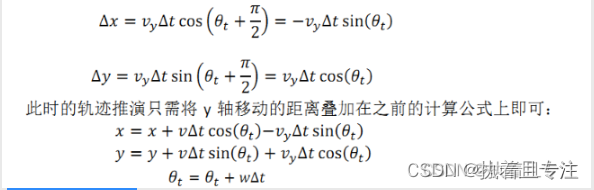### 2、速度采样

（1）自身最大速度最小速度的限制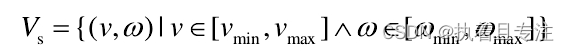（2）受电机性能的影响:由于电机力矩有限，存在最大的加減速限制, 移动机器人轨迹前向模拟的周期内,存在一个动态窗口，该窗口内是机器人能够实际达到的速度（ps: 为啥叫动态窗口）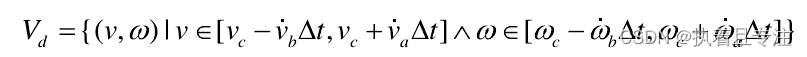（3）安全的考虑：为了能在碰到障碍物前停下, 在最大减速度条件下，速度有一个范围。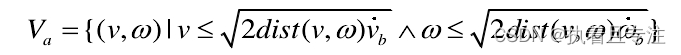### 3、目标函数

一般考虑三种约束，可根据实际进行设计复杂的目标函数：

目标函数 = w1* 方位角函数（轨迹终点朝向与目标点之间的角度差距） + w2* 障碍物函数（轨迹终点位置时与地图上最近障碍物的距离） + w3* 线速度函数（鼓励快速到达终点）

## 三、应用场景

计算复杂度低： 只考虑安全的轨迹，每次采样的时间较短，可以实时避障，但避障效果一般
应用模型： 适用于两轮差分和全向移动模型、不能用在阿克曼模型。
缺点：
（1）前瞻性不足： 只模拟并评价了下一步，如在机器人前段遇见“C”字形障碍时，不能很好的避障
（2）非全局最优路径： 每次都选择下一步的最佳路径，而非全局最优路径

## 四、ros功能包

机器人获得目的地信息后，首先全局路径规划规划出一条大致可行的路线，然后局部路径规划器根据这条路线及costmap的信息规划出机器人在局部时做出具体行动策略，ROS中主要是使用了DWA算法。在ROS中每当move_base处于规划状态就调用DWA算法计算出一条最佳的速度指令，发送给机器人运动底盘执行。
ros导航功能包https://www.guyuehome.com/5500

内容来源于网络和参考链接整理，侵权联系删。

欢迎关注！

展开全文机器学习 人工智能
• 一、DWA算法介绍 DWA算法全称为：Dynamic Window Approach，中文为动态窗口法，其原理主要是在速度空间（v,w）中采样多组速度，并模拟这些速度在一定时间内的运动轨迹，再通过一个评价函数对这些轨迹打分，最优的...

# 一、DWA算法介绍

DWA算法全称为：Dynamic Window Approach，中文为动态窗口法，其原理主要是在速度空间（v,w）中采样多组速度，并模拟这些速度在一定时间内的运动轨迹，再通过一个评价函数对这些轨迹打分，最优的速度被选择出来发送给下位机。
动态窗口的意思是
根据移动机器人的加减速性能限定速度采样空间在一个可行的动态范围呢。

# 二、DWA算法推导

推导过程请参考：
https://blog.csdn.net/heyijia0327/article/details/44983551添加链接描述

## 2.1、建立模型

在上述文章中建立了两种模型：

### 1、模型一

机器人为全向运动，即机器人可以在x，y方向运动，也可以旋转。
则在Δt时间呢，机器人运动轨迹如下：
∙ x = x + v x Δ t cos ⁡ ( θ t ) − v y Δ t sin ⁡ ( θ t ) \bullet x=x+v_{x}\Delta t\cos (\theta_{t} )-v_{y}\Delta t\sin (\theta_{t} )
∙ y = y + v x Δ t sin ⁡ ( θ t ) − v y Δ t cos ⁡ ( θ t ) \bullet y=y+v_{x}\Delta t\sin (\theta_{t} )-v_{y}\Delta t\cos (\theta_{t} )
∙ θ t = θ t + w Δ t \bullet \theta _{t}=\theta _{t}+w\Delta t
其中x，y为世界坐标系， v x v_{x} v y v_{y} 为车辆坐标系，具体如下图所示：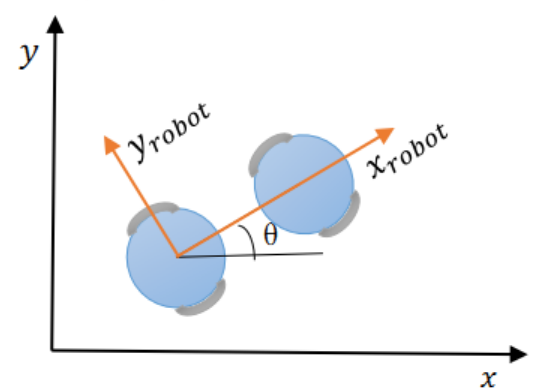### 2、模型二

在模型一中是假设机器人在相邻时间段内的轨迹是直线，模型二采用圆弧代替。
假设机器人不是全向机器人，它做圆弧的半径为：
r = v w r=\frac{v}{w}
当旋转w不为0时，机器人的坐标为：
∙ x = x − v w sin ⁡ ( θ t ) + v w sin ⁡ ( θ t + w Δ t ) \bullet x=x-\frac{v}{w}\sin (\theta _{t})+\frac{v}{w}\sin (\theta _{t}+w\Delta t)
∙ y = y − v w cos ⁡ ( θ t ) − v w cos ⁡ ( θ t + w Δ t ) \bullet y=y-\frac{v}{w}\cos (\theta _{t})-\frac{v}{w}\cos (\theta _{t}+w\Delta t)
∙ θ t = θ t + w Δ t \bullet \theta _{t}=\theta _{t}+w\Delta t

## 2.2、速度采样

根据机器人的轨迹模型，根据速度就可以推算出轨迹，因此只需要采样很多速度，推算轨迹，然后评价这些轨迹好不好即可。
在机器人速度（v,w）的二维空间里存在无限种可能，但是机器人本身和环境的限制可以将机器人的速度限制在一定范围内。主要受以下条件限制：

### 一、移动机器人自身最大最小速度限制

V m = { v ∈ [ v m i n , v m a x ] , w ∈ [ w m i n , w m a x ] } V_{m}=\left \{v\in \left [ v_{min},v_{max}\right ],w\in \left [ w_{min},w_{max}\right ]\right \}

### 二、移动机器人受电机性能影响

由于电机扭矩有限，因此存在最大加减速，因此存在一个动态窗口，在窗口内的速度就是机器人可以达到的最大速度。
V d = { ( v , w ) ∣ v ∈ [ v c − v ˙ b Δ t , v c + v ˙ a Δ t ] w ∈ [ w c − w ˙ b Δ t , w c + w ˙ a Δ t ] } v ˙ V_{d}=\left \{(v,w)|\begin{matrix} v\in \left [ v_{c}-\dot{v}_{b}\Delta t,v_{c}+\dot{v}_{a}\Delta t\right ]\\ w\in \left [ w_{c}-\dot{w}_{b}\Delta t,w_{c}+\dot{w}_{a}\Delta t\right ] \end{matrix}\right \}\dot{v}
其中， v c v_{c} , w c w_{c} 是机器人的当前速度， v ˙ b \dot{v}_{b} v ˙ a \dot{v}_{a} w ˙ b \dot{w}_{b} w ˙ a \dot{w}_{a} 分别对应最大加速度，最大减速度。

### 三、基于移动机器人安全考虑

为了能在碰到障碍物之前停下，因此在最大减速度范围下，速度有一个范围：
V a = { ( v , w ) ∣ v ≤ 2 ⋅ d i s t ( v , w ) ⋅ v ˙ b , w ≤ 2 ⋅ d i s t ( v , w ) ⋅ w ˙ b } V_{a}=\left \{(v,w)|v\leq \sqrt{2\cdot dist(v,w)\cdot \dot{v}_{b}},w\leq \sqrt{2\cdot dist(v,w)\cdot \dot{w}_{b}}\right \}
其中， d i s t ( v , w ) dist(v,w) 为速度 ( v , w ) (v,w) 对应轨迹上离障碍物最近的距离，如下图所示：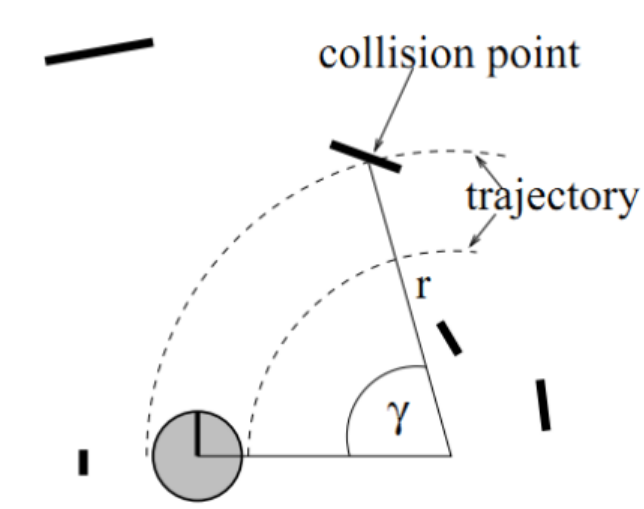注意：这个条件需要模拟出轨迹，找到障碍物位置之后，计算出障碍物到轨迹的距离，然后看当前速度能否在碰到障碍物之前停下，如果能停下，这个速度就是可接受的，否则，这对速度就得抛弃。

## 2.3、评价函数

在采样的速度组中，有若干组轨迹是可行的，因此采用评价函数的方式对每条轨迹进行评价，采用的评价函数如下:
G ( v , w ) = σ ( α ⋅ h e a d i n g ( v , w ) + β ⋅ d i s t ( v , w ) + γ ⋅ v e l o c i t y ( v , w ) ) G(v,w)=\sigma (\alpha \cdot heading(v,w)+\beta \cdot dist(v,w)+\gamma \cdot velocity(v,w))

### 一、方位角评价函数

h e a d i n g ( v , w ) heading(v,w) 是用来评价机器人在当前设定的采样速度下，达到模拟轨迹末端时的朝向和目标之间的角度差距，如下图所示：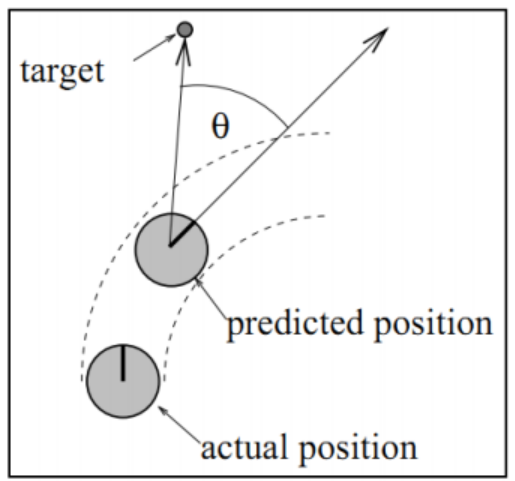### 二、空隙

d i s t ( v , w ) dist(v,w) 代表机器人在当前轨迹上与最近的障碍物之间的距离。如果这条轨迹上没有障碍物，那就将其设定为一个常数。

### 三、速度

v e l o c i t y ( v , w ) velocity(v,w) 用来评价当前轨迹的速度大小。

### 四、平滑处理

上面三个部分计算出来后，每一个需要进行归一化处理，之后再相加。
即每一项除以每一项的总和：
n o r m a l − h e a d ( i ) = h e a d ( i ) ∑ n i = 1 h e a d ( i ) normal_{-}head(i)=\frac{head(i)}{\sum_{n}^{i=1}head(i)}
n o r m a l − d i s t ( i ) = d i s t ( i ) ∑ n i = 1 d i s t ( i ) normal_{-}dist(i)=\frac{dist(i)}{\sum_{n}^{i=1}dist(i)}
n o r m a l − v e l o c i t y ( i ) = v e l o c i t y ( i ) ∑ n i = 1 v e l o c i t y ( i ) normal_{-}velocity(i)=\frac{velocity(i)}{\sum_{n}^{i=1}velocity(i)}
其中，n为采样的所有轨迹点，i为待评价的当前轨迹点。

# 三、DWA算法实现

程序中代码注释已经很全，也可以参考以下内容：
https://blog.csdn.net/subiluo/article/details/81912732

% -------------------------------------------------------------------------
%
% File : DynamicWindowApproachSample.m
%
% Discription : Mobile Robot Motion Planning with Dynamic Window Approach
%
% Environment : Matlab
%
% Author : Atsushi Sakai
%
% Copyright (c): 2014 Atsushi Sakai
%
% License : Modified BSD Software License Agreement
% log: 20181031 增加详细的注释信息 下标的定义
% 20181101 ：增加画出障碍物的大小，更直观的看到障碍物和机器人之间的位置关系
% -------------------------------------------------------------------------

function [] = DynamicWindowApproachSample()

close all;
clear all;

disp('Dynamic Window Approach sample program start!!')

% x=[0 0 pi/2 0 0]'; % 5x1矩阵 列矩阵  位置 0，0 航向 pi/2 ,速度、角速度均为0
x = [0 0 pi/10 0 0]';

POSE_X      = 1;  %坐标 X
POSE_Y      = 2;  %坐标 Y
YAW_ANGLE   = 3;  %机器人航向角
V_SPD       = 4;  %机器人速度
W_ANGLE_SPD = 5;  %机器人角速度

goal = [10,10];   % 目标点位置 [x(m),y(m)]

% 障碍物位置列表 [x(m) y(m)]
obstacle=[0 2;
2 4;
2 5;
4 2;
%           4 4;
5 4;
%           5 5;
5 6;
5 9
8 8
8 9
7 9];
% obstacle=[0 2;
%           4 2;
%           4 4;
%           5 4;
%           5 5;
%           5 6;
%           5 9
%           8 8
%           8 9
%           7 9
%           6 5
%           6 3
%           6 8
%           6 7
%           7 4
%           9 8
%           9 11
%           9 6];

obstacleR = 0.5;% 冲突判定用的障碍物半径
global dt;
dt = 0.1;% 时间[s]

% 机器人运动学模型参数
%定义Kinematic的下标含义
MD_MAX_V    = 1;%   最高速度m/s]
MD_MAX_W    = 2;%   最高旋转速度[rad/s]
MD_ACC      = 3;%   加速度[m/ss]
MD_VW       = 4;%   旋转加速度[rad/ss]
MD_V_RESOLUTION  = 5;%  速度分辨率[m/s]
MD_W_RESOLUTION  = 6;%  转速分辨率[rad/s]]

% 航向得分的比重、距离得分的比重、速度得分的比重、向前模拟轨迹的时间
evalParam = [0.05, 0.2 ,0.1, 3.0];

area      = [-1 11 -1 11];% 模拟区域范围 [xmin xmax ymin ymax]

% 模拟实验的结果
result.x=[];   %累积存储走过的轨迹点的状态值
tic; % 估算程序运行时间开始

% movcount=0;
%% Main loop   循环运行 5000次 指导达到目的地 或者 5000次运行结束
for i = 1:5000
% DWA参数输入 返回控制量 u = [v(m/s),w(rad/s)] 和 轨迹
[u,traj] = DynamicWindowApproach(x,Kinematic,goal,evalParam,obstacle,obstacleR);
x = f(x,u);% 机器人移动到下一个时刻的状态量 根据当前速度和角速度推导 下一刻的位置和角度

% 历史轨迹的保存
result.x = [result.x; x'];  %最新结果 以列的形式 添加到result.x

% 是否到达目的地
if norm(x(POSE_X:POSE_Y)-goal')<0.5   % norm函数来求得坐标上的两个点之间的距离
disp('Arrive Goal!!');break;
end

%====Animation====
hold off;               % 关闭图形保持功能。 新图出现时，取消原图的显示。
ArrowLength = 0.5;      % 箭头长度

% 机器人
% quiver(x,y,u,v) 在 x 和 y 中每个对应元素对组所指定的坐标处将向量绘制为箭头
quiver(x(POSE_X), x(POSE_Y), ArrowLength*cos(x(YAW_ANGLE)), ArrowLength*sin(x(YAW_ANGLE)), 'ok'); % 绘制机器人当前位置的航向箭头
hold on;                                                     %启动图形保持功能，当前坐标轴和图形都将保持，从此绘制的图形都将添加在这个图形的基础上，并自动调整坐标轴的范围

plot(result.x(:,POSE_X),result.x(:,POSE_Y),'-b');hold on;    % 绘制走过的所有位置 所有历史数据的 X、Y坐标
plot(goal(1),goal(2),'*r');hold on;                          % 绘制目标位置

%plot(obstacle(:,1),obstacle(:,2),'*k');hold on;              % 绘制所有障碍物位置
DrawObstacle_plot(obstacle,obstacleR);

% 探索轨迹 画出待评价的轨迹
if ~isempty(traj) %轨迹非空
for it=1:length(traj(:,1))/5    %计算所有轨迹数  traj 每5行数据 表示一条轨迹点
ind = 1+(it-1)*5; %第 it 条轨迹对应在traj中的下标
plot(traj(ind,:),traj(ind+1,:),'-g');hold on;  %根据一条轨迹的点串画出轨迹   traj(ind,:) 表示第ind条轨迹的所有x坐标值  traj(ind+1,:)表示第ind条轨迹的所有y坐标值
end
end

axis(area); %根据area设置当前图形的坐标范围，分别为x轴的最小、最大值，y轴的最小最大值
grid on;
drawnow;  %刷新屏幕. 当代码执行时间长，需要反复执行plot时，Matlab程序不会马上把图像画到figure上，这时，要想实时看到图像的每一步变化情况，需要使用这个语句。
%movcount = movcount+1;
%mov(movcount) = getframe(gcf);%  记录动画帧
end
toc  %输出程序运行时间  形式：时间已过 ** 秒。
%movie2avi(mov,'movie.avi');  %录制过程动画 保存为 movie.avi 文件

%% 绘制所有障碍物位置
% 输入参数：obstacle 所有障碍物的坐标   obstacleR 障碍物的半径
function [] = DrawObstacle_plot(obstacle,obstacleR)
r = obstacleR;
theta = 0:pi/20:2*pi;
for id=1:length(obstacle(:,1))
x = r * cos(theta) + obstacle(id,1);
y = r  *sin(theta) + obstacle(id,2);
plot(x,y,'-m');hold on;
end
% plot(obstacle(:,1),obstacle(:,2),'*m');hold on;              % 绘制所有障碍物位置

%% DWA算法实现
% 输入参数：当前状态、模型参数、目标点、评价函数的参数、障碍物位置、障碍物半径
% 返回参数：控制量 u = [v(m/s),w(rad/s)] 和 轨迹集合 N * 31  （N：可用的轨迹数）
% 选取最优参数的物理意义：在局部导航过程中，使得机器人避开障碍物，朝着目标以较快的速度行驶。
function [u,trajDB] = DynamicWindowApproach(x,model,goal,evalParam,ob,R)
% Dynamic Window [vmin,vmax,wmin,wmax] 最小速度 最大速度 最小角速度 最大角速度速度
Vr = CalcDynamicWindow(x,model);  % 根据当前状态 和 运动模型 计算当前的参数允许范围

% 评价函数的计算 evalDB N*5  每行一组可用参数 分别为 速度、角速度、航向得分、距离得分、速度得分
%               trajDB      每5行一条轨迹 每条轨迹都有状态x点串组成
[evalDB,trajDB]= Evaluation(x,Vr,goal,ob,R,model,evalParam);  %evalParam 评价函数参数 [heading,dist,velocity,predictDT]

if isempty(evalDB)
disp('no path to goal!!');
u=[0;0];return;
end

% 各评价函数正则化
evalDB = NormalizeEval(evalDB);

% 最终评价函数的计算
feval=[];
for id=1:length(evalDB(:,1))
feval = [feval;evalParam(1:3)*evalDB(id,3:5)']; %根据评价函数参数 前三个参数分配的权重 计算每一组可用的路径参数信息的得分
end
evalDB = [evalDB feval]; % 最后一组

[maxv,ind] = max(feval);% 选取评分最高的参数 对应分数返回给 maxv  对应下标返回给 ind
u = evalDB(ind,1:2)';% 返回最优参数的速度、角速度

%% 评价函数 内部负责产生可用轨迹
% 输入参数 ：当前状态、参数允许范围（窗口）、目标点、障碍物位置、障碍物半径、评价函数的参数
% 返回参数：
%           evalDB N*5  每行一组可用参数 分别为 速度、角速度、航向得分、距离得分、速度得分
%           trajDB      每5行一条轨迹 每条轨迹包含 前向预测时间/dt + 1 = 31 个轨迹点（见生成轨迹函数）
function [evalDB,trajDB] = Evaluation(x,Vr,goal,ob,R,model,evalParam)
evalDB = [];
trajDB = [];
for vt = Vr(1):model(5):Vr(2)       %根据速度分辨率遍历所有可用速度： 最小速度和最大速度 之间 速度分辨率 递增
for ot=Vr(3):model(6):Vr(4)     %根据角度分辨率遍历所有可用角速度： 最小角速度和最大角速度 之间 角度分辨率 递增
% 轨迹推测; 得到 xt: 机器人向前运动后的预测位姿; traj: 当前时刻 到 预测时刻之间的轨迹（由轨迹点组成）
[xt,traj] = GenerateTrajectory(x,vt,ot,evalParam(4),model);  %evalParam(4),前向模拟时间;
% 各评价函数的计算
dist    = CalcDistEval(xt,ob,R);    % 前项预测终点 距离最近障碍物的间隙得分 距离越远分数越高
vel     = abs(vt);                  % 速度得分 速度越快分越高
stopDist = CalcBreakingDist(vel,model); % 制动距离的计算
if dist > stopDist % 如果可能撞到最近的障碍物 则舍弃此路径 （到最近障碍物的距离 大于 刹车距离 才取用）
evalDB = [evalDB;[vt ot heading dist vel]];
trajDB = [trajDB;traj];   % 每5行 一条轨迹
end
end
end

%% 归一化处理
% 每一条轨迹的单项得分除以本项所有分数和
function EvalDB=NormalizeEval(EvalDB)
% 评价函数正则化
if sum(EvalDB(:,3))~= 0
EvalDB(:,3) = EvalDB(:,3)/sum(EvalDB(:,3));  %矩阵的数除  单列矩阵的每元素分别除以本列所有数据的和
end
if sum(EvalDB(:,4))~= 0
EvalDB(:,4) = EvalDB(:,4)/sum(EvalDB(:,4));
end
if sum(EvalDB(:,5))~= 0
EvalDB(:,5) = EvalDB(:,5)/sum(EvalDB(:,5));
end

%% 单条轨迹生成、轨迹推演函数
% 输入参数： 当前状态、vt当前速度、ot角速度、evaldt 前向模拟时间、机器人模型参数（没用到）
% 返回参数;
%           x   : 机器人模拟时间内向前运动 预测的终点位姿(状态);
%           traj: 当前时刻 到 预测时刻之间 过程中的位姿记录（状态记录） 当前模拟的轨迹
%                  轨迹点的个数为 evaldt / dt + 1 = 3.0 / 0.1 + 1 = 31
%
function [x,traj] = GenerateTrajectory(x,vt,ot,evaldt,model)
global dt;
time = 0;
u = [vt;ot];% 输入值
traj = x;   % 机器人轨迹
while time <= evaldt
time = time+dt; % 时间更新
x = f(x,u);     % 运动更新 前项模拟时间内 速度、角速度恒定
traj = [traj x]; % 每一列代表一个轨迹点 一列一列的添加
end

%% 计算制动距离
%根据运动学模型计算制动距离, 也可以考虑成走一段段圆弧的累积 简化可以当一段段小直线的累积
function stopDist = CalcBreakingDist(vel,model)
global dt;
MD_ACC   = 3;%
stopDist=0;
while vel>0   %给定加速度的条件下 速度减到0所走的距离
stopDist = stopDist + vel*dt;% 制动距离的计算
vel = vel - model(MD_ACC)*dt;%
end

%% 障碍物距离评价函数  （机器人在当前轨迹上与最近的障碍物之间的距离，如果没有障碍物则设定一个常数）
% 输入参数：位姿、所有障碍物位置、障碍物半径
% 输出参数：当前预测的轨迹终点的位姿距离所有障碍物中最近的障碍物的距离 如果大于设定的最大值则等于最大值
% 距离障碍物距离越近分数越低
function dist = CalcDistEval(x,ob,R)
dist=100;
for io = 1:length(ob(:,1))
disttmp = norm(ob(io,:)-x(1:2)')-R; %到第io个障碍物的距离 - 障碍物半径  ！！！有可能出现负值吗？？
if dist > disttmp   % 大于最小值 则选择最小值
dist = disttmp;
end
end

% 障碍物距离评价限定一个最大值，如果不设定，一旦一条轨迹没有障碍物，将太占比重
if dist >= 2*R %最大分数限制
dist = 2*R;
end

% 输入参数：当前位置、目标位置
% 输出参数：航向参数得分  当前车的航向和相对于目标点的航向 偏离程度越小 分数越高 最大180分
theta = toDegree(x(3));% 机器人朝向
goalTheta = toDegree(atan2(goal(2)-x(2),goal(1)-x(1)));% 目标点相对于机器人本身的方位
if goalTheta > theta
targetTheta = goalTheta-theta;% [deg]
else
targetTheta = theta-goalTheta;% [deg]
end

heading = 180 - targetTheta;

%% 计算动态窗口
% 返回 最小速度 最大速度 最小角速度 最大角速度速度
function Vr = CalcDynamicWindow(x,model)

V_SPD       = 4;%机器人速度
W_ANGLE_SPD = 5;%机器人角速度

MD_MAX_V = 1;%
MD_MAX_W = 2;%
MD_ACC   = 3;%
MD_VW    = 4;%

global dt;
% 车子速度的最大最小范围 依次为：最小速度 最大速度 最小角速度 最大角速度速度
Vs=[0 model(MD_MAX_V) -model(MD_MAX_W) model(MD_MAX_W)];

% 根据当前速度以及加速度限制计算的动态窗口  依次为：最小速度 最大速度 最小角速度 最大角速度速度
Vd = [x(V_SPD)-model(MD_ACC)*dt x(V_SPD)+model(MD_ACC)*dt x(W_ANGLE_SPD)-model(MD_VW)*dt x(W_ANGLE_SPD)+model(MD_VW)*dt];

% 最终的Dynamic Window
Vtmp = [Vs;Vd];  %2 X 4  每一列依次为：最小速度 最大速度 最小角速度 最大角速度速度
Vr = [max(Vtmp(:,1)) min(Vtmp(:,2)) max(Vtmp(:,3)) min(Vtmp(:,4))];

%% Motion Model 根据当前状态推算下一个控制周期（dt）的状态
% u = [vt; wt];当前时刻的速度、角速度 x = 状态[x(m),y(m),yaw(Rad),v(m/s),w(rad/s)]
function x = f(x, u)
global dt;
F = [1 0 0 0 0
0 1 0 0 0
0 0 1 0 0
0 0 0 0 0
0 0 0 0 0];

B = [dt*cos(x(3)) 0
dt*sin(x(3)) 0
0 dt
1 0
0 1];

x= F*x+B*u;

%% degree to radian

%% radian to degree
function degree = toDegree(radian)

%% END

展开全文• 此部分内容是ROS中Move_Base功能包用到的DWA路径规划算法的介绍和实现，下面我将以自己所理解的DWA算法原理内容展示出来，我看过网上和书籍很多资料，它们的描述基本大同小异，对于想要清楚了解或刚接触DWA算法的人...

## 前言

此部分内容是ROS中Move_Base功能包用到的DWA路径规划算法的介绍和实现，下面我将以自己所理解的DWA算法原理内容展示出来，我看过网上和书籍很多资料，它们的描述基本大同小异，对于想要清楚了解或刚接触DWA算法的人来讲真的比较难理解可能要多看那么四五遍或五六遍才能真正理解这也是比较耗费时间的，所以本文在我理解的基础上写的，本文内容如果有不当之处还请谅解，如果有错误之处也请指出，毕竟本文是以我自己所理解的方式去完成的。

一、DWA算法原理
首先先给出DWA算法的原理流程图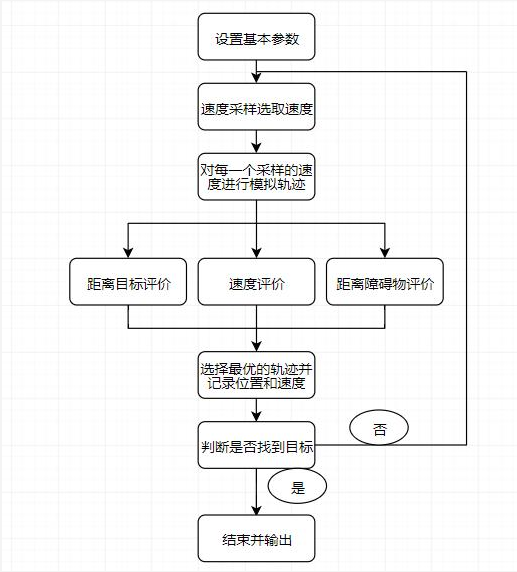首先我将先讲一讲评价函数的计算，评价函数其实是比较简单的。
1、距离目标点评价函数：距离目标点评价函数这里采用的就是很普遍也很普通的欧式距离算法，但具体是哪两个点的距离呢，目标点是人为一开始给定的，我们已经有了，另一个点就是一个速度的模拟轨迹的最后一个位置，可能看到这里还会比较模糊什么是模拟轨迹的最后一个位置，没关系，这里先说一下评价函数要求对评价函数怎么算心中有个概念即可。
2、速度评价函数：速度评价函数也比较简单，就是计算刚开始人为设定的机器最大线速度值和模拟轨迹的最后一个位置的线速度的差值，总而言之就是两个速度差值就是，这里也是一样先有个概念。
3、轨迹距离障碍物的评价函数：轨迹距离障碍物也比较简单，就是对一条轨迹上每个采样的位置进行循环，再对每个障碍物进行循环，也就是一条模拟轨迹路线上每个位置和每个障碍物之间的欧氏距离如何，然后比较得出一个距离最小的值，当然在循环的过程中要不断判断每个距离是不是小于机器人自身半径，因为如果小于自身半径我们判定机器人已经撞上去了这时候就直接返回。

其次，我们要开始讲一下速度采样的问题了，这里我要偷一张图来帮助理解了。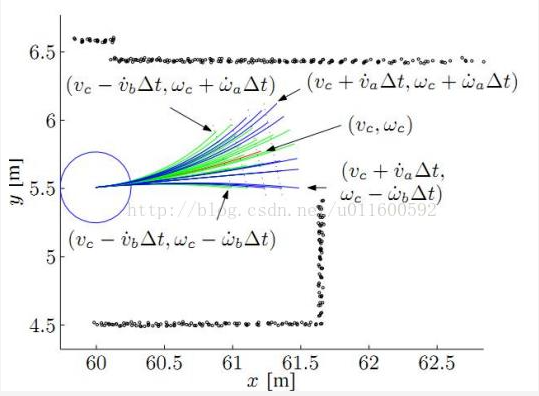之后速度采样完后我们就可以开始计算模拟轨迹上各个点的位置等相关信息了。
位置的计算其实很简单就是如下图计算公式：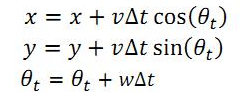这里的v就是线速度，w就是角速度，这里可能很多人会好奇为什么这里计算位置使用直线方式计算，这是因为我们一条模拟轨迹上所设定的时间间隔很小所以可以近似把它看作直线来计算，同时这也使得代码计算更为方便快捷，那这里我再偷一张图。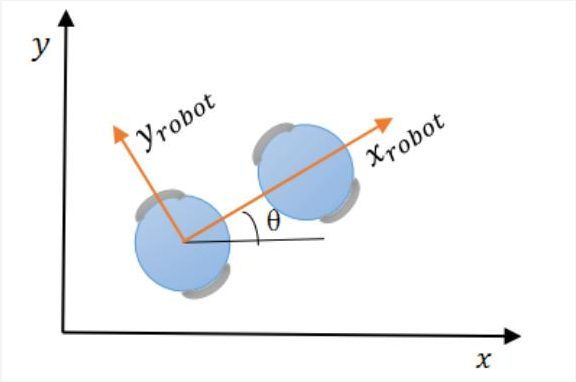这里可能大家会看到机器人还有个Yrobot方向速度，大家其实可以不用管，因为一般正常的轮子都是只能实现前后方向的位置改变，但是可能有些人会接触到麦克纳姆轮也就是全向移动轮，它可以做到前后左右位置改变，所以其实这里我们大可不必考虑Y方向速度，如果你真想知道你就在百度上随便搜一篇DWA算法你就会在里面找到相关计算方法，但是这里我并不想阐述计算方法。

最后，讲到这里DWA算法基本的东西其实已经全部讲完了，其实这个算法并不会太难，如果看不懂就多读几遍，最后的步骤就是不断地循环循环找最优轨迹并沿着最优轨迹走然后再速度采样再模拟轨迹再选择最优轨迹走，直到到达某个位置和目标点的距离不超过自己设定的范围为止。对了，刚刚上面只讲了评价函数但是我给忘了讲评价分的计算，其实也很简单，最简单的方法就是把三个评价函数计算出来的结果全部相加起来当然这是最简单的计算方法，实际上我们不能这样，因为每个参量有大有小，可能因为某个评价函数的结果过大直接覆盖了其他两个，所以我们还是要进行平滑处理的操作，这里我要再去偷个公式图。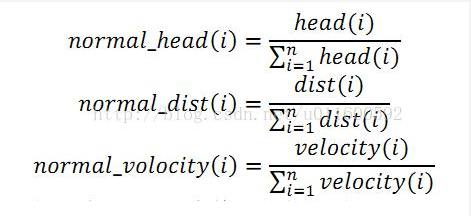二、DWA算法Python实现代码
首先说明一下这个代码我是参考给出的标准的DWA算法实现，但是我是在自己完全理解后自己看着DWA公式来写出算法，代码的每一步基本都有注释。
这份代码里面可能大家看到，我没有用权重系数和归一化，这是因为我是在坐标轴上一个小区域直接模拟，所以计算量都不是很大，所以我就没有用到，在真正以后实际情况里，还是要按照公式来做

import numpy as np
from math import *
import matplotlib.pyplot as plt

#参数设置
V_Min = -0.5            #最小速度
V_Max = 3.0             #最大速度
W_Min = -50*pi/180.0    #最小角速度
W_Max = 50*pi/180.0     #最大角速度
Va = 0.5                #加速度
Wa = 30.0*pi/180.0      #角加速度
Vreso = 0.01            #速度分辨率
Wreso = 0.1*pi/180.0    #角速度分辨率
radius = 1              #机器人模型半径
Dt = 0.1                #时间间隔
Predict_Time = 4.0      #模拟轨迹的持续时间
alpha = 1.0             #距离目标点的评价函数的权重系数
Belta = 1.0             #速度评价函数的权重系数
Gamma = 1.0             #距离障碍物距离的评价函数的权重系数

#障碍物
Obstacle=np.array([[0,10],[2,10],[4,10],[6,10],[3,5],[4,5],[5,5],[6,5],[7,5],[8,5],[10,7],[10,9],[10,11],[10,13]])
#Obstacle = np.array([[0, 2]])

#距离目标点的评价函数
def Goal_Cost(Goal,Pos):
return sqrt((Pos[-1,0]-Goal)**2+(Pos[-1,1]-Goal)**2)

#速度评价函数
def Velocity_Cost(Pos):
return V_Max-Pos[-1,3]

#距离障碍物距离的评价函数
def Obstacle_Cost(Pos,Obstacle):
MinDistance = float('Inf')          #初始化时候机器人周围无障碍物所以最小距离设为无穷
for i in range(len(Pos)):           #对每一个位置点循环
for j in range(len(Obstacle)):  #对每一个障碍物循环
Current_Distance = sqrt((Pos[i,0]-Obstacle[j,0])**2+(Pos[i,1]-Obstacle[j,1])**2)  #求出每个点和每个障碍物距离
if Current_Distance < radius:            #如果小于机器人自身的半径那肯定撞到障碍物了返回的评价值自然为无穷
return float('Inf')
if Current_Distance < MinDistance:
MinDistance=Current_Distance         #得到点和障碍物距离的最小

return 1/MinDistance

#速度采用
def V_Range(X):
Vmin_Actual = X-Va*Dt          #实际在dt时间间隔内的最小速度
Vmax_actual = X+Va*Dt          #实际载dt时间间隔内的最大速度
Wmin_Actual = X-Wa*Dt          #实际在dt时间间隔内的最小角速度
Wmax_Actual = X+Wa*Dt          #实际在dt时间间隔内的最大角速度
VW = [max(V_Min,Vmin_Actual),min(V_Max,Vmax_actual),max(W_Min,Wmin_Actual),min(W_Max,Wmax_Actual)]  #因为前面本身定义了机器人最小最大速度所以这里取交集
return VW

#一条模拟轨迹路线中的位置，速度计算
def Motion(X,u,dt):
X+=u*dt*cos(X)           #x方向上位置
X+=u*dt*sin(X)           #y方向上位置
X+=u*dt                     #角度变换
X=u                         #速度
X=u                         #角速度
return X

#一条模拟轨迹的完整计算
def Calculate_Traj(X,u):
Traj=np.array(X)
Xnew=np.array(X)
time=0
while time <=Predict_Time:        #一条模拟轨迹时间
Xnew=Motion(Xnew,u,Dt)
Traj=np.vstack((Traj,Xnew))   #一条完整模拟轨迹中所有信息集合成一个矩阵
time=time+Dt
return Traj

#DWA核心计算
def dwa_Core(X,u,goal,obstacles):
vw=V_Range(X)
best_traj=np.array(X)
min_score=10000.0                 #随便设置一下初始的最小评价分数
for v in np.arange(vw, vw, Vreso):         #对每一个线速度循环
for w in np.arange(vw, vw, Wreso):     #对每一个角速度循环
traj=Calculate_Traj(X,[v,w])
goal_score=Goal_Cost(goal,traj)
vel_score=Velocity_Cost(traj)
obs_score=Obstacle_Cost(traj,Obstacle)
score=goal_score+vel_score+obs_score
if min_score>=score:                    #得出最优评分和轨迹
min_score=score
u=np.array([v,w])
best_traj=traj

return u,best_traj

x=np.array([2,2,45*pi/180,0,0])                          #设定初始位置，角速度，线速度
u=np.array([0,0])                                        #设定初始速度
goal=np.array([8,8])                                     #设定目标位置
global_tarj=np.array(x)
for i in range(1000):                                     #循环1000次，这里可以直接改成while的直到循环到目标位置
u,current=dwa_Core(x,u,goal,Obstacle)
x=Motion(x,u,Dt)
global_tarj=np.vstack((global_tarj,x))                 #存储最优轨迹为一个矩阵形式每一行存放每一条最有轨迹的信息
print('Arrived')
break

plt.plot(global_tarj[:,0],global_tarj[:,1],'*r',Obstacle[0:3,0],Obstacle[0:3,1],'-g',Obstacle[4:9,0],Obstacle[4:9,1],'-g',Obstacle[10:13,0],Obstacle[10:13,1],'-g')  #画出最优轨迹的路线
plt.show()

效果展示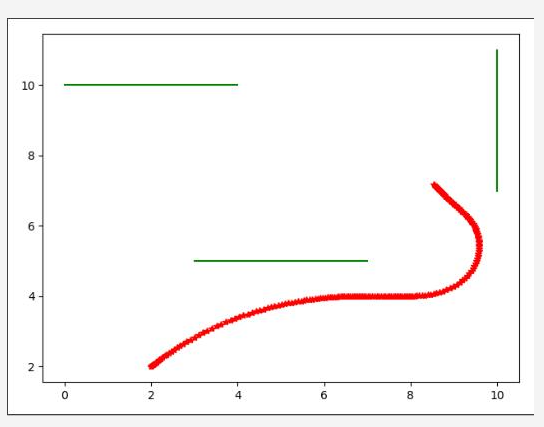三、结语和总结
其实看不懂的东西只要多看几遍多耐心的琢磨就肯定会明白其中的道理，多看几遍真的没有错。只要自己不要自暴自弃就好，以前老师总说不懂的知识多看几遍，我一直不放在心上，长大后才发现老师说的真的是对的，很多事情也是如此，多看多做几遍，简单的事情重复做，重复的事情坚持做！

四、参考文献
https://blog.csdn.net/u011600592/article/details/54613717
https://scm_mos.gitlab.io/motion-planner/dwa-local-planner/

展开全文DWA
• 2021SC@SDUSC ROS源代码阅读（9） 后面主要看dwa算法
• DWA算法的核心： DWA的核心在于所谓的动态窗口，这个东西在sampling based method 中就是sampling. 对于sampling可以是去sample state，也可以sample action. 百度的lattice planner其实就是在sample state, 把未来...
• ## DWA算法分析

万次阅读 多人点赞 2019-08-17 13:39:47
机器人在获得目的地信息后，首先经过全局路径规划规划出一条大致可行的路线，然后调用局部路径规划器根据这条路线及costmap的信息规划出机器人在局部时做出具体行动策略，ROS中主要是使用了DWA算法。在ROS中每当...
• 前言 此部分内容是ROS中Move_Base功能包用到的DWA路径规划算法的介绍和实现，下面我将以自己所理解的DWA算法原理内容展示出来，我看过网上和书籍很多资料，它们的描述基本大同小异
• ## DWA算法原理

千次阅读 2019-05-28 10:36:44
DWA算法 局部路径规划简介 机器人在获得目的地信息后，首先经过全局路径规划规划出一条大致可行的路线，然后调用局部路径规划器根据这条路线及costmap的信息规划出机器人在局部时做出具体行动策略，ROS中主要是使用...
• % 绘制所有障碍物位置 %% DWA算法实现 % model 机器人运动学模型 最高速度m/s],最高旋转速度[rad/s],加速度[m/ss],旋转加速度[rad/ss], 速度分辨率[m/s],转速分辨率[rad/s]] % 输入参数：当前状态、模型参数、目标...
• ## DWA算法详解

万次阅读 2019-05-30 11:05:27
DWA (robotPose,robotGoal,robotModel) laserscan = readScanner() allowable_v = generateWindow(robotV, robotModel) allowable_w = generateWindow(robotW, robotModel) for each v in allowable_v for ...
• 【路径规划】基于改进动态窗口DWA算法机器人静态避障matlab源码.zip
• 自己写的船舶避碰用的动态避碰仿真，可直接运行，参数可调
• dwa算法简介 动态窗口法(dynamic window approach, dwa)，用于实现机器人的局部路径规划 实现原理： 在速度空间(v,w)中不断采样，模拟机器人在采样得到的速度下的运行轨迹，并针对这些轨迹进行评价，从而选取最优的...
• DWA算法全称为dynamic window approach，其原理主要是在速度空间（v,w）中采样多组速度，并模拟这些速度在一定时间内的运动轨迹，再通过一个评价函数对这些轨迹打分，最优的速度被选择出来发送给下位机。 1 原理分析...matlab
• ROS的路径规划器分为全局路径和局部路径规划，其中局部路径规划器使用的最广的为dwa，个人理解为： 首先全局路径规划会生成一条大致的全局路径，局部路径规划器会把全局路径给分段，然后根据分段的全局路径的坐标，...DWA
• ## 自动驾驶路径规划DWA算法原理解析

万次阅读 多人点赞 2019-09-25 22:50:36
这篇文章详细介绍一下最新的ROS给出的DWA算法的结构，以及各个重要的cost function的含义，帮助你们理解DWA算法的构成。决策规划 动态规划
• DWA算法是基于机器人运动学与动力学理论的一种局部避障算法，它将对机器人的位置控制转换为对机器人的速度控制。DWA算法可以概括为三步:一是根据机器人自身的限制以及环境制约将速度的采样空间约束在一定范围内; 二...matlab
• ## DWA算法

千次阅读 2017-07-07 19:35:32
http://blog.csdn.net/heyijia0327/article/details/44983551
• ## DWA路径规划算法

千次阅读 2022-06-21 00:43:59
来源丨古月居1.DWA路径规划基本原理动态窗口法主要是在速度(v,w)空间中...该算法突出点在于动态窗口这个名词，它的含义是依据移动机器人的加减速性能限定速度采样空间在一个可行的动态范围内。2.DWA路径规划流程3....python 机器学习 人工智能 java
• 一、DWA算法简介 DWA算法全称为dynamic window approach，其原理主要是在速度空间（v,w）中采样多组速度，并模拟这些速度在一定时间内的运动轨迹，再通过一个评价函数对这些轨迹打分，最优的速度被选择出来发送给下...matlab
• 本篇博客将重点介绍DWA算法所采用的评价函数中与参考路径相关的评价函数 评价函数: 轨迹主要依据以下三条准则进行评分,综合评分后选取分数最小的路径作为下一时刻选择路径: Obstacle_costs 轨迹上是否存在......